+

# Straight Line Depreciation

Author: Sophia Tutorial
##### Description:

Calculate the straight line depreciation for an asset.

(more)### Developing Effective Teams

*No strings attached. This college course is 100% free and is worth 1 semester credit.

37 Sophia partners guarantee credit transfer.

299 Institutions have accepted or given pre-approval for credit transfer.

* The American Council on Education's College Credit Recommendation Service (ACE Credit®) has evaluated and recommended college credit for 32 of Sophia’s online courses. Many different colleges and universities consider ACE CREDIT recommendations in determining the applicability to their course and degree programs.

Tutorial
what's covered
This lesson will cover the topic of straight line depreciation.

Our discussion breaks down as follows:

1. Straight Line Depreciation
2. Straight Line Depreciation Calculation
3. Straight Line Depreciation Examples

## 1. Straight Line Depreciation

With straight line depreciation, we are assuming that the life of the asset is assumed to be used up as a constant rate over its useful life. Straight line depreciation should be used if there is consistent usage of the asset over the asset's useful life, meaning there are no large fluctuations, increases or decreases, in terms of the usage level of that asset over time.

EXAMPLE

For example, buildings aren't necessarily used more in the early years and less in the later years of their lifespan. Generally speaking, they are used consistently over their useful life.

A major benefit of straight line depreciation is simplicity. The asset is depreciated in equal dollar amounts over the useful life of the asset; therefore, it's going to be the same depreciation amount every year. This significantly simplifies the depreciation calculation that needs to be performed.

## 2. Straight Line Depreciation Calculation

The calculation for a straight line depreciation is as follows: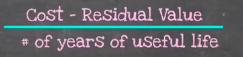The numerator, when you're calculating straight line depreciation, is cost minus residual value, and the denominator is the number of years of the asset's useful life.

Now, let's break down this calculation further into its component parts:.

• Cost refers to the cost to purchase, acquire, or build the asset.
• Residual value is the estimated value of an asset at the end of its useful life.

hint
Residual value represents any value that is left over once you've reached the end of the useful life of the asset, so you can potentially sell that used asset for a sum of money equal to its estimated residual value.

The straight line depreciation calculation provides an annual amount. Note, we can also break it down into monthly depreciation by dividing our calculated straight line depreciation amount by 12. In addition, if we're looking for the amount of accumulated depreciation as of a certain month, we would simply multiply that monthly amount by the number of months that we're looking for.

term to know
Residual Value
The estimated value of an asset at the end of its useful life
formula
Straight Line Depreciation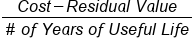## 3. Straight Line Depreciation Examples

Next, let's take a look at our straight line depreciation formula by performing some calculations with some example scenarios. For each example, we'll need to know the total cost, residual value, as well as the asset's useful life. You can also see we have input our straight line depreciation formula: cost minus residual value divided by the number of years of the useful life.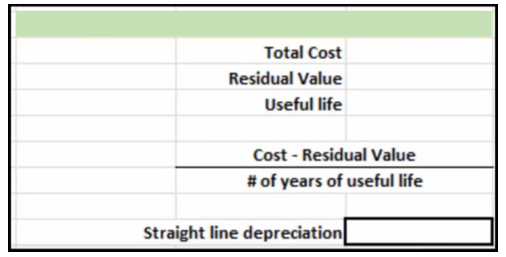Example #1
For the first example, the total cost of the asset is \$500,000, and we're going to assume it has a residual value of \$15,000 and a useful life of 10 years. Plugging this information into our formula gives us a straight line depreciation of \$48,500.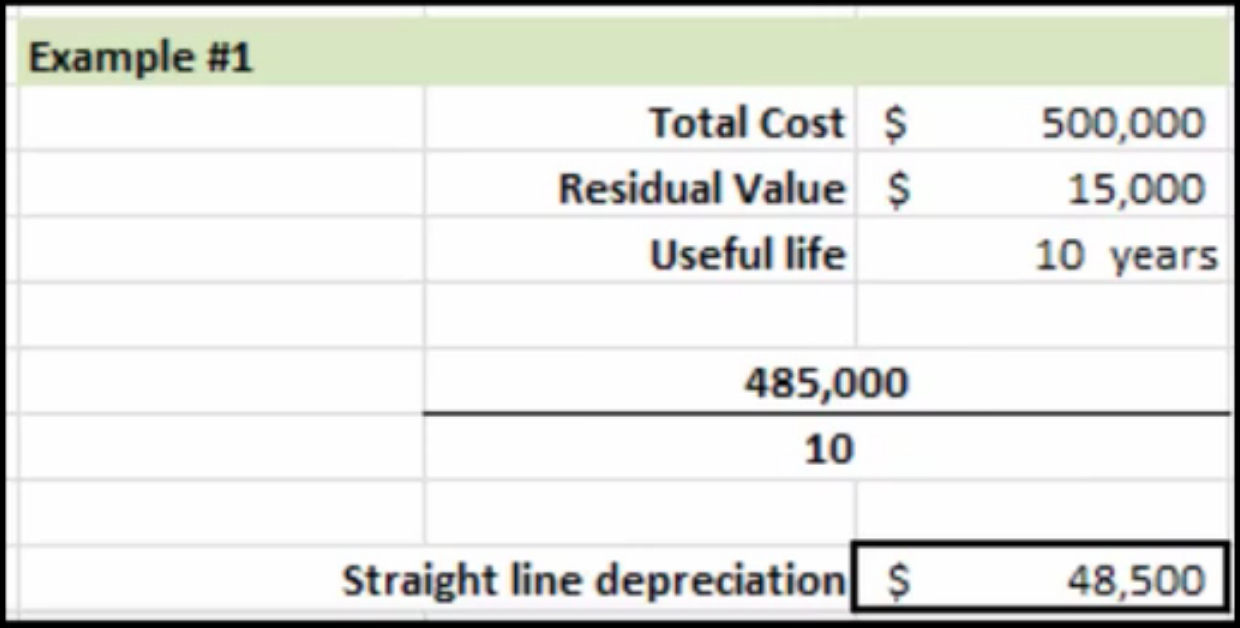Example #2
For the second example, we're going to assume we have a total cost of \$1,000,000, a residual value of \$50,000, and a useful life of 15 years. We can plug this information into our straight line depreciation formula to give us our straight line depreciation, or annual depreciation, of 63,333.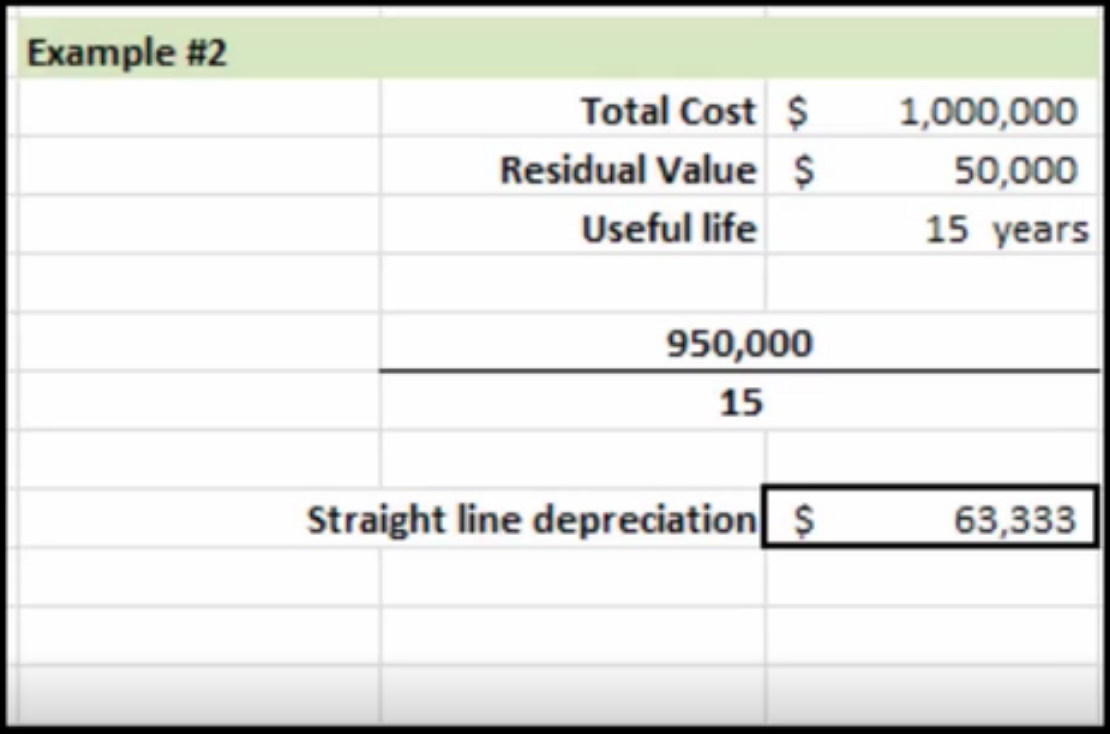Now, we can break that down even further by looking at the monthly depreciation.

In this case, every month, depreciation would be \$5,278.

If we wanted to see what the accumulated depreciation would be at a certain point in time, we can take that monthly depreciation amount and multiply it by the number of months that we want to find. In this example, after 36 months, the total accumulated depreciation would be \$190,008, which is the monthly depreciation multiplied by 36.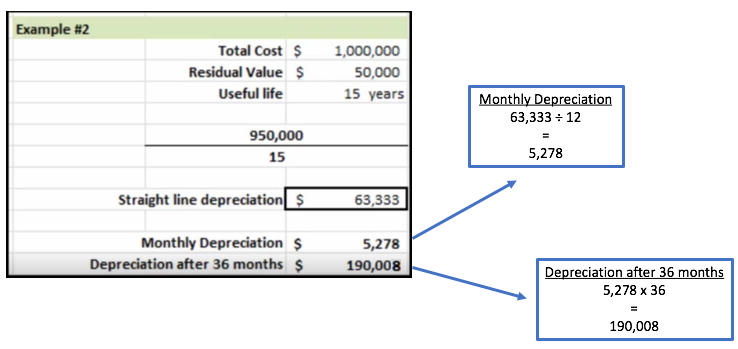summary
Today we learned all about straight line depreciation, including the formula for straight line depreciation calculation: cost minus residual value divided by the number of years of useful life. Lastly, we looked at some examples of performing the straight line depreciation calculation.

Source: Adapted from Sophia instructor Evan McLaughlin.

Terms to Know
Residual value

The estimated value of an asset at the end of its useful life.

Rating